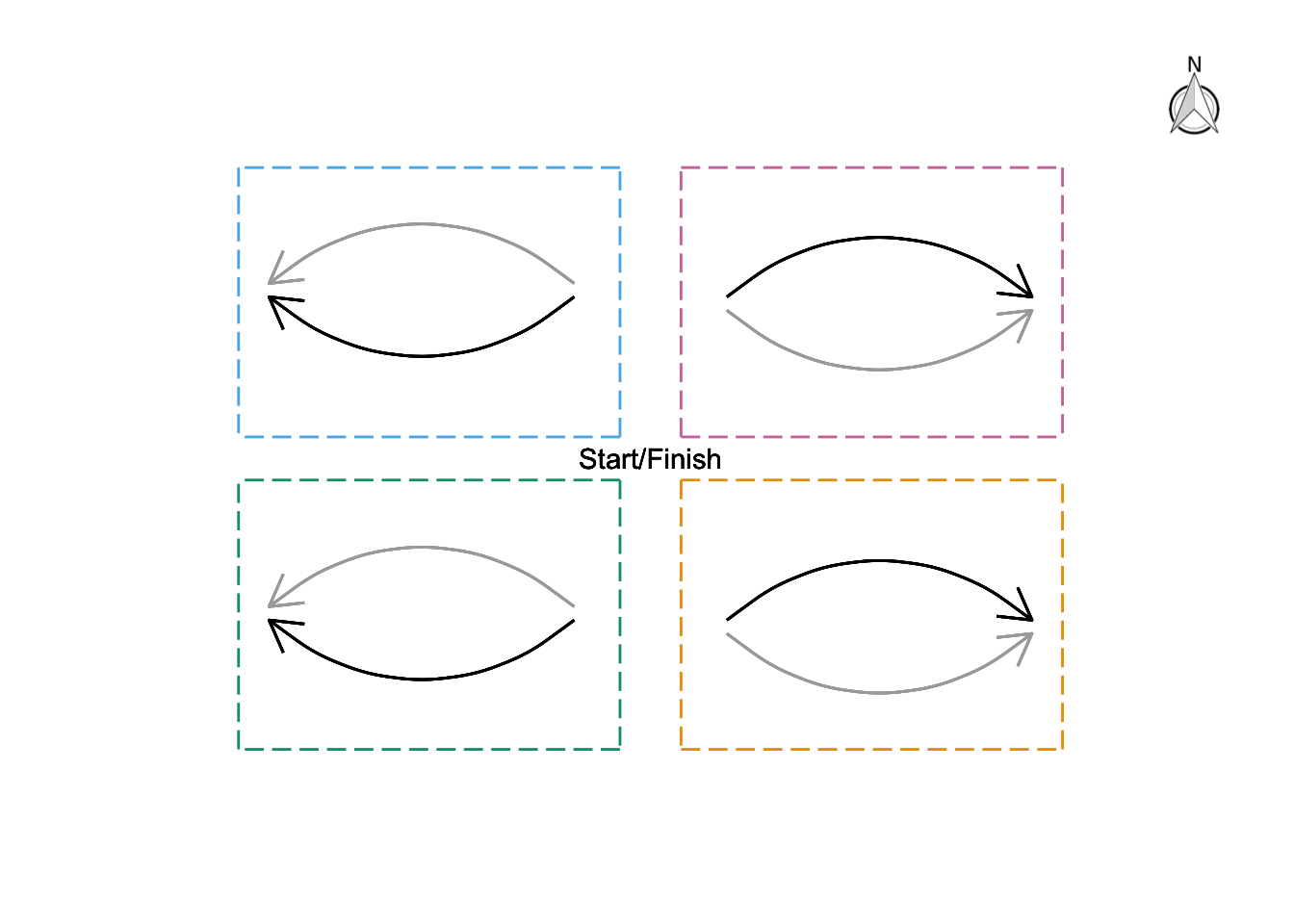# Puzzle from the New Scientist

Attempt to solve #115 “A random robot” puzzle from the New Scientist. This week (No 3336 - 29 May 2021) the New Scientist published the following puzzle:

Roman the test robot is being given one final roam before being consigned to the scrapheap where he can rust in peace.

He has been programmed to make four equal length steps. For his first move, he can travel one step east, west, north or south. Each of his subsequent three steps must be at right angles to the previous move. The direction of each move is selected by a random number generator, with all four possibilities being equally probable.

What is the chance that Roman will finish where he started?

I decided to solve the puzzle by first plotting how (and whether) Roman can finish where he started (see graph below). To do this, I divided the space based on the 4 cardinal directions he can take in one step. These are the coloured quadrants in the plot; his Start/Finish position is also given. For example, Roman will be travelling along the purple top-right quadrant if he starts by going either north or east.

Next, within each quadrant he can travel clockwise or anti-clockwise. This is depicted with the black and grey arrows, respectively. For instance, if he goes north at the first step (purple quadrant) and then by travelling clockwise (black arrow), at right angles, he will finish where he started. Similarly, if he goes east at the first step (purple quadrant) and then by travelling anti-clockwise (grey arrow) he will again finish where he started. In effect, the purple quadrant corresponds to the path:

north -> east -> south -> west

or

east -> north -> west -> southUsing the same reasoning, within each quadrant Roman can travel clockwise or anti-clockwise. This gives us 8 different paths (4 quadrants times 2 directions in each one) in total for Roman to start and finish at the same position.

Now we need to calculate the probability of each of those paths. I’ll use the path:

north -> east -> south -> west

for illustration. For his first move, he can choose any of the four cardinal directions with equal probability. So, the probability he chooses north is 1/4 (i.e. P(north)=1/4). After that, for each subsequent step he can only travel at right angels to the previous move. This limits his choices to two at each subsequent step. In addition, either choice is equally probable (as given by the problem). In this example, after going north, he can only go east or west with equal probability, so P(east) = 1/2. The same is valid of the next two steps. Hence, P(south) = 1/2 and P(west) = 1/2. To find the probability of the entire path we simply need to multiply the individual probabilities of the path. This is, P(path) = P(north) * P(east) * P(south) * P(west) = 1/32.

Finally, we have 8 different paths so the total probability that Roman will finish where he started is 8*(1/32)=1/4.

## Update 04/06/21

Indeed, the answer is 1/4, see here for the New Scientist’s solution.